The Australian Journal of Mathematical Analysis and Applications

 Home News Editors Volumes RGMIA Subscriptions Authors Contact

ISSN 1449-5910

You searched for grandville
Total of 17 results found in site

6: Paper Source PDF document

Paper's Title:

A New Property of General Means of Order p with an Application to the Theory of Economic Growth

Author(s):

Olivier de La Grandville

Department of Management Science and Engineering,
Huang Engineering Center, Stanford University,
475 Via Ortega, Stanford, California 94305
U.S.A.

lagrandvil@aol.com

Abstract:

The purpose of this note is to demonstrate a new property of the general mean of order p of m ordered positive numbers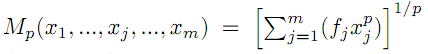. If p < 0 and if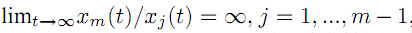, the elasticity of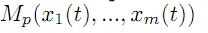with respect to xm, defined by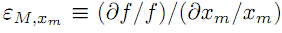, tends towards zero, and therefore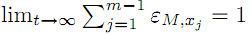. This property is then applied to optimal growth theory.

3: Paper Source PDF document

Paper's Title:

End-Point and Transversality Conditions in the Calculus of Variations: Derivations through Direct Reasoning

Author(s):

Olivier de La Grandville

Stanford University,
Department of Management Science and Engineering,
475 Via Ortega, Stanford, CA 94305,
U. S. A.

E-mail: ola@stanford.edu

Abstract:

We offer an intuitive explanation of the end-point and transversality conditions that complement the Euler equation in the calculus of variations. Our reasoning is based upon the fact that any variation given to an optimal function must entail a zero net gain to the functional, all consequences of implied changes in its derivative being fully taken into account.

3: Paper Source PDF document

Paper's Title:

Introducing the Dorfmanian: A Powerful Tool for the Calculus Of Variations

Author(s):

Olivier de La Grandville

Department of Management Science and Engineering,
Stanford University,
475 Via Ortega, Stanford, CA 94305,
U. S. A.

E-mail: odelagrandville@gmail.com

Abstract:

We show how a modified Hamiltonian proposed by Robert Dorfman  to give intuitive sense to the Pontryagin maximum principle can be extended to easily obtain all high-order equations of the calculus of variations. This new concept is particularly efficient to determine the differential equations leading to the extremals of functionals defined by n-uple integrals, while a traditional approach would require -- in some cases repeatedly -- an extension of Green's theorem to n-space.
Our paper is dedicated to the memory of Robert Dorfman (1916 - 2002).

3: Paper Source PDF document

Paper's Title:

A New Interpretation of the Number e

Author(s):

Olivier de La Grandville

Faculty of Economics, Goethe University,
60323 Frankfurt am Main,
Germany.
E-mail: odelagrandville@gmail.com

Abstract:

We show that e is the amount that 1 becomes when it is invested during an arbitrary time span of length T, at any continuously compounded interest rates as long as their average is equal to 1/T . A purely mathematical interpretation of e is the amount a unit quantity becomes after any duration T when the average of its instantaneous growth rates is 1/T. This property can be shown to remain valid if T tends to infinity as long as the integral of the growth rates converges to unity.

2: Paper Source PDF document

Paper's Title:

A One-Line Derivation of the Euler and Ostrogradski Equations

Author(s):

Olivier de La Grandville

Stanford University,
Department of Management Science and Engineering,
Stanford, CA 94305,
U. S. A

Abstract:

At the very heart of major results of classical physics, the Euler and Ostrogradski equations have apparently no intuitive interpretation. In this paper we show that this is not so. Relying on Euler's initial geometric approach, we show that they can be obtained through a direct reasoning that does not imply any calculation. The intuitive approach we suggest offers two benefits: it gives immediate significance to these fundamental second-order non-linear differential equations; and second, it allows to obtain a property of the calculus of variations that does not seem to have been uncovered until now: the Euler and Ostrogradski equations can be derived not necessarily by giving a variation to the optimal function -- as is always done; one could equally well start by giving a variation to their derivative(s).

Search and serve lasted 0 second(s).